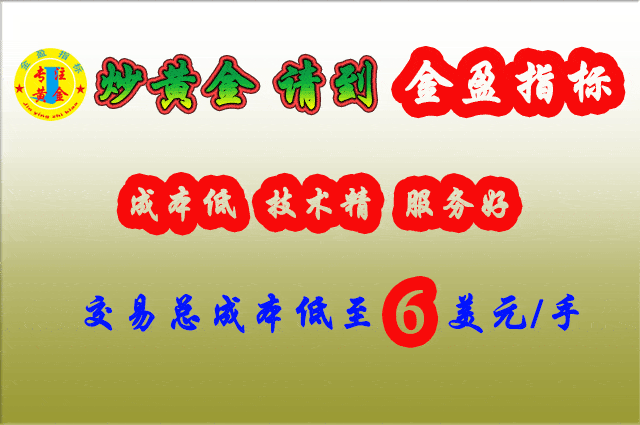# 现货金|常用技术指标-黄金分割线 毛利率分析“金线”的使用

10.00=10×1.000

13.82=10×1.382

15=10×1.500

16.18=10×1.618

20.00=10×2.000

26.18=10×2.618

6.18=10×0.618

5=10×0.5

3.82=10×0.382

1.91=10×0.191

1.从回调幅度来看

2.从反弹幅度来看